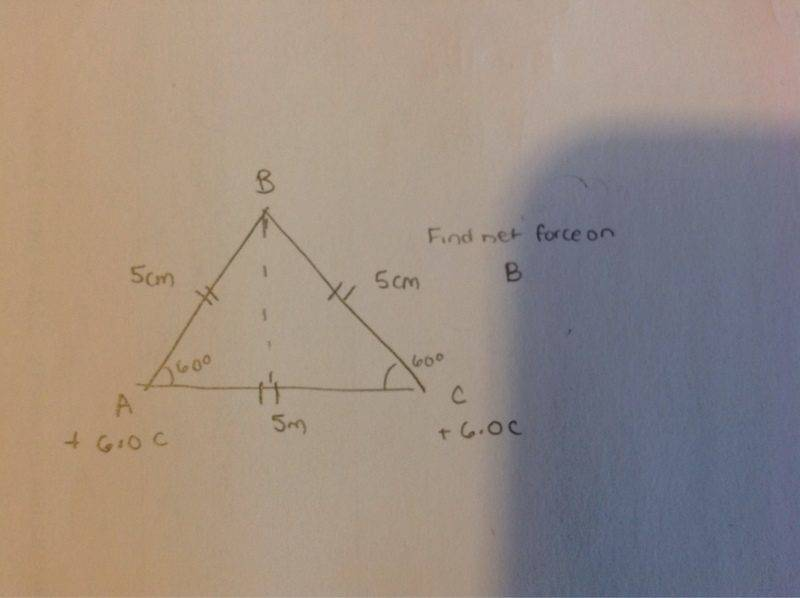# Equilateral triangle net force

• Coco12

## Homework Statement

Lets say I have an equilateral triangle, and you are asked to calculate the net force on the top of the triangle

Coulombs Law

## The Attempt at a Solution

I know to use Coulombs law to find the forces acting on the top of the triangle, then since it is an equilateral triangle, the angle would be 60 degrees and you use that to find the x and y components of the forces acting on the top.
The x components of the the resultant would cancel out = 0
The y components would be some number. Then you would just use pythagorean theorem to find the overall net force. However when you find the direction: Tan inverse of Ry/Rx

Rx would be 0 therefore it is undefined. Does that mean that the direction of the net force on the top of the triangle would be 0 degrees?

Seems like your problem statement is missing some information. Why are you using Coulomb's Law? Are there some unmentioned charges attached to this triangle?

Seems like your problem statement is missing some information. Why are you using Coulomb's Law? Are there some unmentioned charges attached to this triangle?

Yes there are. The spheres are all negatively charged and have the same charge

I used coulombs law because it asked the find the magnitude and direction of the net electric force.

And what is the permittivity of the turtle's shell?

And what is the permittivity of the turtle's shell?

Ok what?

Does anyone have any other input?

You have not provided enough information for anyone to understand what you are talking about.

And see this FAQ.U use columbs law for a on b and con b , then you draw it out on a Cartesian plane
Next you find the x and y components of each.
Then you add them together. The x components cancel out